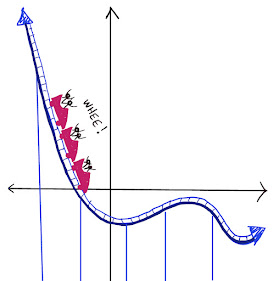## Saturday, August 13, 2016

### Balance Puzzles

The scales below are balanced.  Each scale is a different puzzle.

For each scale, start by taking off as many blocks as you can from each side (if any) while keeping the scale balanced.

Once you do, assume one blue ball weighs 12 grams. What is the mass of the other shapes?

For the solution, click "Read More" below.

Solution:

1.    This one is not possible to "reduce." But we have enough information to solve.  If a blue ball is 12 grams, then a green square is 24 grams.

2.    We can reduce this by removing a triangle from each side (the addition/subtraction property of equality).  We end up with a blue ball on one side and a pink triangle on the other, so they are equal. Both are 12 grams.

3.    Here we can divide both sides by 2 (the multiplication/division property of equality), and we are left with one blue ball equal to two triangles.  If the blue ball has a mass of 12, then the triangles each have a mass of 6.

4.    Here, let's start by subtracting a blue ball from each side.  We then have two blue balls equal to four pink triangles.  Then divide both sides by 2. So one blue ball equal to two triangles.  If the blue ball has a mass of 12, then the triangles each have a mass of 6.

5.    Start by subtracting an orange rectangle and a pink triangle from both sides. We have one blue ball equal to one orange triangle, so each one has a mass of 12.  In this case it is not possible to tell the mass of the pink triangles.

6.    Start by subtracting an orange rectangle from both sides. We have three blue balls equal to six orange rectangles.  Divide both sides by 3.  Now we have one blue ball equal to two orange triangles.  So if the blue ball has a mass of 12, then the orange triangle has a mass of 6.

7.    Start by subtracting four orange rectangles, one blue ball, and one red triangle from each side.  We are left with one blue ball equal to two orange rectangles. If the blue ball is worth 12, then the orange rectangles are each worth 6.  In this case it is not possible to tell the mass of the red triangles.

8.    Start by subtracting one blue ball, two orange rectangles, and one star from both sides.  We are left with two blue balls equal to six stars.  Divide both by 2.  A blue ball (weighing 12 grams) is equal to 3 stars (weighing 4 each).

#### 1 comment:

1.